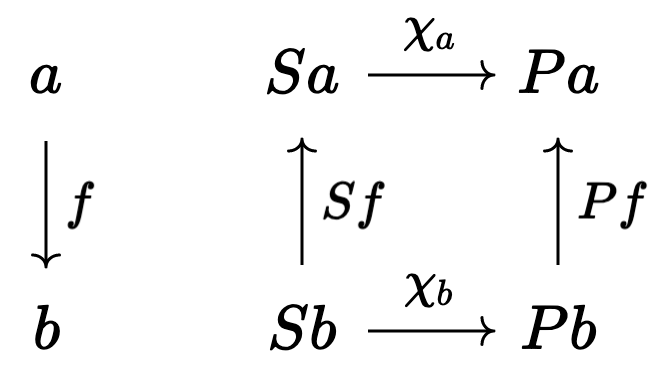# Merlin's Notebook

A blog by Benjamin Merlin Bumpus

# Understanding Sheaves §3

In a previous post, we discussed sieves, the sieve-y definition of Grothendieck topologies and a few exaples thereof. Today we’ll return to sheaves, but this time we do so armed with a better understanding of sites (the “places” in which to define sheaves).

This post consists of notes and reflections from reading Daniel Rosiak’s book “Sheaf Theory through Examples“.

## A refresher

As you might recall, the frist definition of a sheaf which we saw was that of a sheaf on the subgraph topology; namely a functor $$\mathcal{F}: \mathbf{Sub}G \to \mathbf{Set}$$ satisfying the condition that, whenever we are given an $$I$$-indexed mathching family of sections $$(s_i \in \mathcal{F}G_i)_{i \in I}$$ (i.e. satisfying the requirement that $$s_i|_{G_i \cap G_j} = s_j|_{G_i \cap G_j}$$ for all $$i$$ and $$j$$), then there is a unique section $$s \in \mathcal{F}\bigl(\bigcup_{i \in I }G_i\bigr)$$ which restrict to each section in the family (i.e. such that $$s|_{G_i} = s_i$$).

Notice that, when one says that a functor is a sheaf, one must also specify with respect to which topology it is a sheaf. For instance, in the definition above, we have that $$\mathcal{F}$$ is a sheaf with respect to the subobject topology on $$\mathbf{Sub}G$$ (but it might fail to be a sheaf with respect to some other topology on $$\mathbf{Sub}G$$. The key point is that, if we change the topology, then we are also changing what it means when we state that a family of sections is a matching family. So the first step forwards is to define the matching condition more generally.

## Matching families

Recal that a sieve $$S$$ on an object $$c \in C$$ can be defined as a subfunctor of the representable $$y_c \colon C \to \mathbf{Set}^{C^{op}}$$ $$y_c \colon b \mapsto C(b, c).$$ You should think of $$S$$ as the functor taking each $$b \in C$$ to the subset set of all morphisms in $$C(b,c)$$ which are in the sieve $$S$$.

Def : Let $$(C, K)$$ be a site, $$S$$ be a $$K$$-cover of an object $$c \in C$$ and $$P: C^{op} \to \mathbf{Set}$$ be a presheaf. Then a matching family of sections of $$P$$ with respect to the cover $$S$$ is a morphism of presheaves $$\chi \colon S \Rightarrow P$$.

Let’s unpack this. Let $$a$$ be an object of $$C$$. The component of $$\chi$$ at $$a$$ is a function $$\chi_a \colon Sa \to Pa$$ which “picks-out” a section in $$Pa$$ for each element of $$Sa$$ (think an “open” in $$S$$). The naturality of $$\chi$$ ensures that every morphism $$f \colon a \to b$$ gives rise to a commutative squarewhich intuitively states, for any “open” $$\beta \in Sb$$ in $$b$$, that “if you restrict $$\beta$$ to $$a$$ (via $$Sf$$) and then consider its associated section $$\chi_a Sf(\beta)$$, you’ll get the same answer as if you had first grabbed ahold of the section $$\chi_b(\beta)$$ associated to $$\beta$$ and then restricted that section to $$a$$ (via $$Pf$$)”. If you compare this to the definition of a matching family on the subgraph topology I gave earlier, you’ll see that the intuition carries over.

Now we’re ready to give a proper definition of a sheaf on a site.

Def: Let $$P \colon C^{op} \to \mathbf{Set}$$ be a presheaf on a site $$(C, K)$$. Then we call $$P$$ a sheaf with respect to $$K$$ (or a $$K$$-sheaf) if $$\forall c \in C \text{ and } \\ \forall \text{ covering sieves } \bigl(\iota \colon S \Rightarrow y_c\bigr) \in Kc \text{ of } c \text{ and } \\ \forall \text{ matching families } \chi: S \Rightarrow P$$ there is a unique extension $$\mathcal{E} \colon y_c \Rightarrow P$$ making the following triangle commute.

Let’s process that definition one step at a time, starting with the quantifiers;. We’re just saying the usual thing: “$$P$$ is a sheaf if there is a unique extension for every matching family $$\chi$$ w.r.t. any cover $$S$$ of any object $$c$$”. That’s the trivial part.

The harder part to unpack is understanding how the morphism of presheaves $$\mathcal{E}_\chi$$ encodes the amalgamation property of sections of sheaves (it’s the usual big equalizer diagram.. see the first post in the series if you don’t remember what I’m talking about). This is not apparent “on the nose”. But it becomes clear once you invoke the Yoneda Lemma: since the natural transformations $$\mathsf{Nat}( y_c , P)$$ from $$y_c$$ to $$P$$ are in one-to-one correspondence with the elements of $$Pc$$, a morphism of presheaves $$\mathcal{E}: y_c \Rightarrow P$$ is just a way of picking an element of $$Pc$$ — i.e. a global section on $$c$$. The fact that the triangle commutes is simply saying that the global section $$\mathcal{E}$$ which we picked must restrict to the local sections picked by the matching family $$\chi$$.

Anyway, today is a short post because I’m taking my Christmas spirit elsewhere. Ciao!